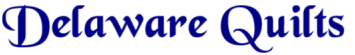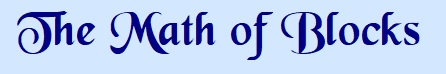One very important thing in making any quilt block is knowing the correct size. You must understand what size you need. If a pattern calls for a 4 inch "finished" diagonal half square, that means the size that square will be when it is completely finished, sewn into the quilt. It does NOT mean the size the square will be when you are finished sewing the two triangles together. Remember this, or your project will never turn out right!

Now what if you find a block you'd like to make but the directions are for a 12 1/2 inch unfinished block and you want to make a nine inch finished block. Let's use the shoofly pattern as an example.

Not to confuse the issue, but ---- The "unfinished" or "raw" block size is 12 1/2 inches. You put it in a quilt, with other blocks, taking up a 1/4 inch seam allowance on each side. That makes the "finished" block, in the quilt, 12 inches. If the finished square is 12 inches, which means the size you see when it is in the quilt.

If you divide those 12 inches into three sections, as for this nine patch shoofly block, each section is 4 inches. (12 divided by 3 = 4) However, you will need seam allowances, so you have to add them. There are two seams in a nine-patch block, and each seam has 1/2 inches of seam allowances (1/4 on each side). So you would add one half inch on each of the three sections to make up your nine patch. (12 divided by 3 = 4 + 1/2 = 4 1/2 inches)

Remember we want a nine inch finished shoofly block, so… we divide 9 inches into three sections, since this shoofly block has three sections, each section is 3 inches. (9 divided by 3 = 3) But we need seam allowances, so we have to add them. There are two seams in a nine-patch block, and each seam has 1/2 inches of seam allowances (1/4 on each side). So you would add one half inch on each of the three sections to make up your nine patch. (9 divided by 3 = 3 + 1/2 = 3 1/2 inches)

Now, for diagonal half squares, the math gets a little more complicated. You just have to remember these few (?) measurements. Remember "finished" sizes, 9 inches. If you are making diagonal half squares to put in a 9 inch "finished" nine-patch square, you will need those diagonal half squares to measure 3 1/2 when you are done. But because your diagonal half squares will have a seam in them, going diagonally from corner to corner, you have to add a seam allowance in them as well. You add 3/8 for this seam allowance. Your squares to make diagonal half squares from should be cut 3 7/8 inches. (3 1/2 + 3/8 = 3 7/8)The same math works if you are making a nine patch block or a 16 patch block, such as Hovering Hawks. We want to make a 12 inch "finished" block with sixteen patches, or four equal sections in each direction. (12 divided by 4 = 3) So we want 3 inch "finished" squares to make up our 16 patch blocks. And we have to add our seam allowances, so we add 1/2. (12 divided by 4 = 3 + 1/2 = 3 1/2 inches) If we are making diagonal half squares, we add 3/8. (3 1/2 + 3/8 = 3 7/8)

|Home|Quilts|Blocks|Retreat| Email|

Updated May 31, 2020